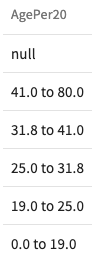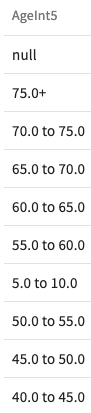Version: 0.32.2

# Bin

`Bin` lets you take the values of a numeric column and group them into bins or buckets of various sizes. Binning can be especially useful for machine learning and predictive analytics to group continuous values into more manageable, or values such as ages, where it might be useful to interpret them as ranges.

### Format​

`Bin` uses the following formats:

• `Bin the column <column> based on percentile setting the number of intervals to <interval count> (<without rounding>) (and call the bins <bin names>)`
• `Bin the column <column> based on width setting the interval size to <size> (and call the bins <bin names>)`
• `Bin the column <column> based on width setting the number of intervals to <interval count> (without rounding) (and call the bins <bin names>)`
• `Bin the column <column> based on width starting the interval values at <values> (and call the bins <bin names>)`

### Parameters​

`Bin` uses the following parameters:

• `column` (required). The numeric column whose values to bin.
• `interval count` (required). The number of bins to create.
• `without rounding` (optional). Use raw values as the bin boundaries. By default, the bin boundaries are rounded to the nearest hundredth.
• `size` (required). The size of each bin.
• `values` (required). A comma-separated list of the starting values for each bin. Note that these values must be listed in increasing order.
• `bin names` (optional). The names to give to the column created by each bin.

### Output​

If the column's values are binned successfully, a success message appears in log. A new dataset is created with a column appended that assigns a bin to each row in the dataset.

Otherwise, an error message appears in the log.

### Examples​

Consider the following column called Age with the following statistics:

• The smallest value is 0.
• The largest value is 80.
• The average age is 30.

To bin the values of this column into percentiles, one bin for every 20%, enter `Bin the column Age based on percentile setting the number of intervals to 5`. Bins like these are created:To bin the values of this column based on width using interval size, one bin for every five years, enter `Bin the column Age based on width setting the interval size to 5`. Bins like these are created:To bin the values of this column based on width with a specific number of bins, enter `Bin the column Age based on width setting the number of intervals to 10`. Bins like these are created:To bin the values of this column using custom bins, such as, 0 to 23, 24 to 32, 33 to 54, and 55+, enter `Bin the column called Age starting the interval values at 0, 24, 33, 55`. Bins like these are created: